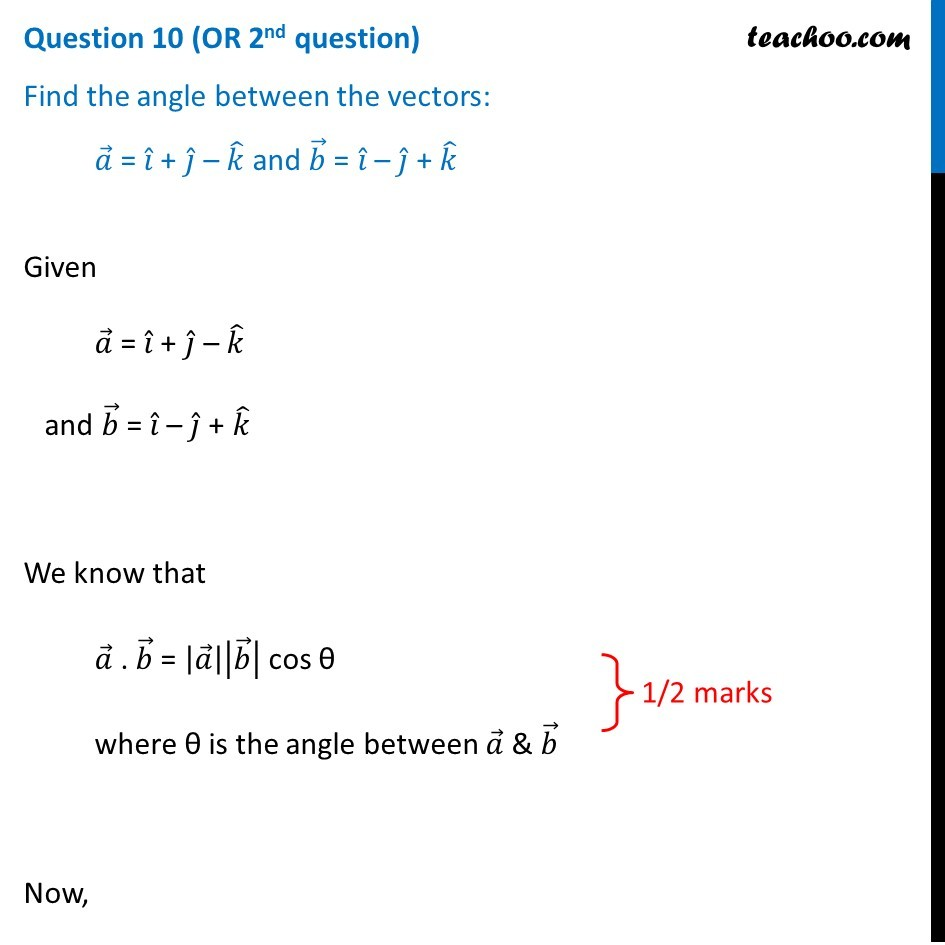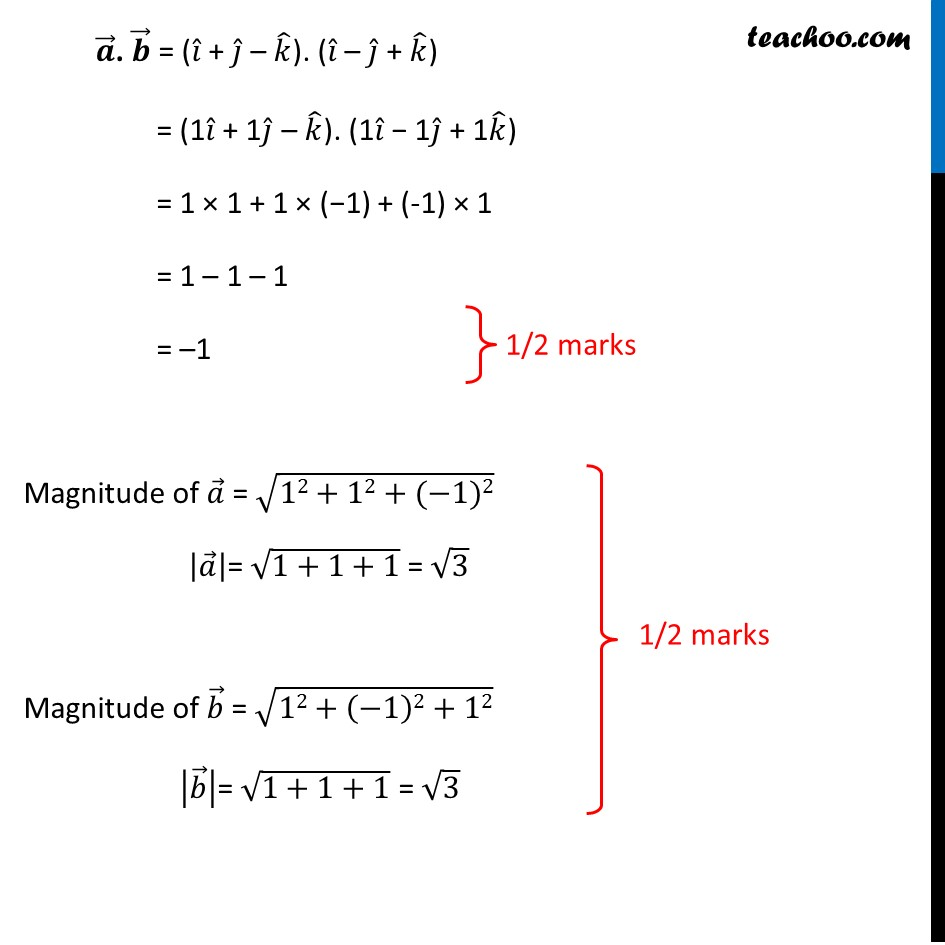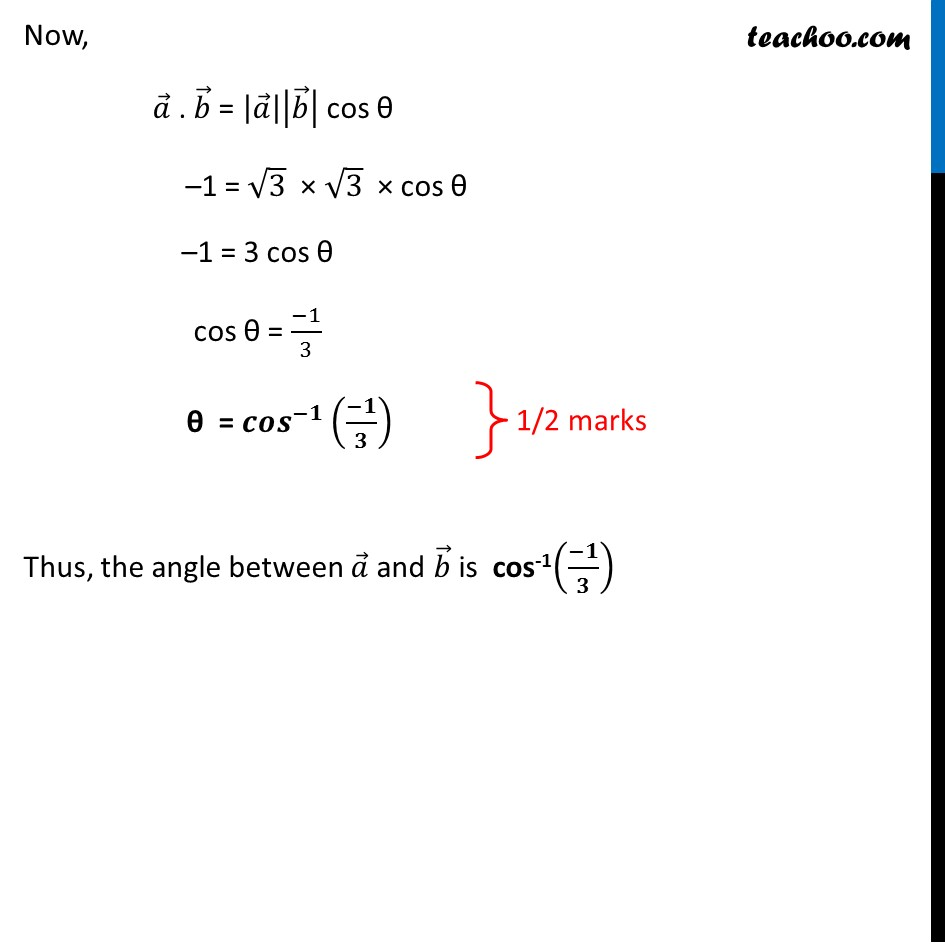CBSE Class 12 Sample Paper for 2019 Boards

Class 12
Solutions of Sample Papers and Past Year Papers - for Class 12 Boards

Question 10 (OR 2 nd question)

Find the angle between the vectors:

a = i + j – k and b = i – j + kLearn in your speed, with individual attention - Teachoo Maths 1-on-1 Class

### Transcript

Question 10 (OR 2nd question) Find the angle between the vectors: 𝑎 ⃗ = 𝑖 ̂ + 𝑗 ̂ – 𝑘 ̂ and 𝑏 ⃗ = 𝑖 ̂ – 𝑗 ̂ + 𝑘 ̂ Given 𝑎 ⃗ = 𝑖 ̂ + 𝑗 ̂ – 𝑘 ̂ and 𝑏 ⃗ = 𝑖 ̂ – 𝑗 ̂ + 𝑘 ̂ We know that 𝑎 ⃗ . 𝑏 ⃗ = |𝑎 ⃗ ||𝑏 ⃗ | cos θ where θ is the angle between 𝑎 ⃗ & 𝑏 ⃗ Now, 𝒂 ⃗. 𝒃 ⃗ = (𝑖 ̂ + 𝑗 ̂ – 𝑘 ̂). (𝑖 ̂ – 𝑗 ̂ + 𝑘 ̂) = (1𝑖 ̂ + 1𝑗 ̂ – 𝑘 ̂). (1𝑖 ̂ − 1𝑗 ̂ + 1𝑘 ̂) = 1 × 1 + 1 × (−1) + (-1) × 1 = 1 – 1 – 1 = –1 Magnitude of 𝑎 ⃗ = √(12+12+(−1)2) |𝑎 ⃗ |= √(1+1+1) = √3 Magnitude of 𝑏 ⃗ = √(12+(−1)2+12) |𝑏 ⃗ |= √(1+1+1) = √3 Now, 𝑎 ⃗ . 𝑏 ⃗ = |𝑎 ⃗ ||𝑏 ⃗ | cos θ –1 = √3 × √3 × cos θ –1 = 3 cos θ cos θ = (−1)/3 θ = 𝒄𝒐𝒔^(−𝟏) ((−𝟏)/𝟑) Thus, the angle between 𝑎 ⃗ and 𝑏 ⃗ is cos-1((−𝟏)/𝟑)